Next: Normalization uncertainty Up: Use and misuse of Previous: Best estimate of the   Contents

### Offset uncertainty

Let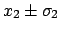and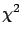be the two measured values, and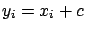the common standard uncertainty: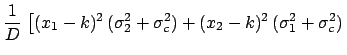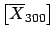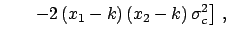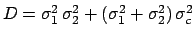(6.48)

where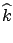is the determinant of the covariance matrix.

Minimizing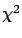and using the second derivative calculated at the minimum we obtain the best value ofand its standard deviation: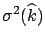(6.49)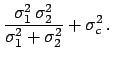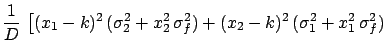(6.50)

The most probable value of the physical quantity is exactly that which one obtains from the average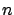weighted with the inverse of the individual variances. Its overall uncertainty is the quadratic sum of the standard deviation of the weighted average and the common one. The result coincides with the simple expectation.Next: Normalization uncertainty Up: Use and misuse of Previous: Best estimate of the   Contents
Giulio D'Agostini 2003-05-15